# Maze generation by site percolation with Python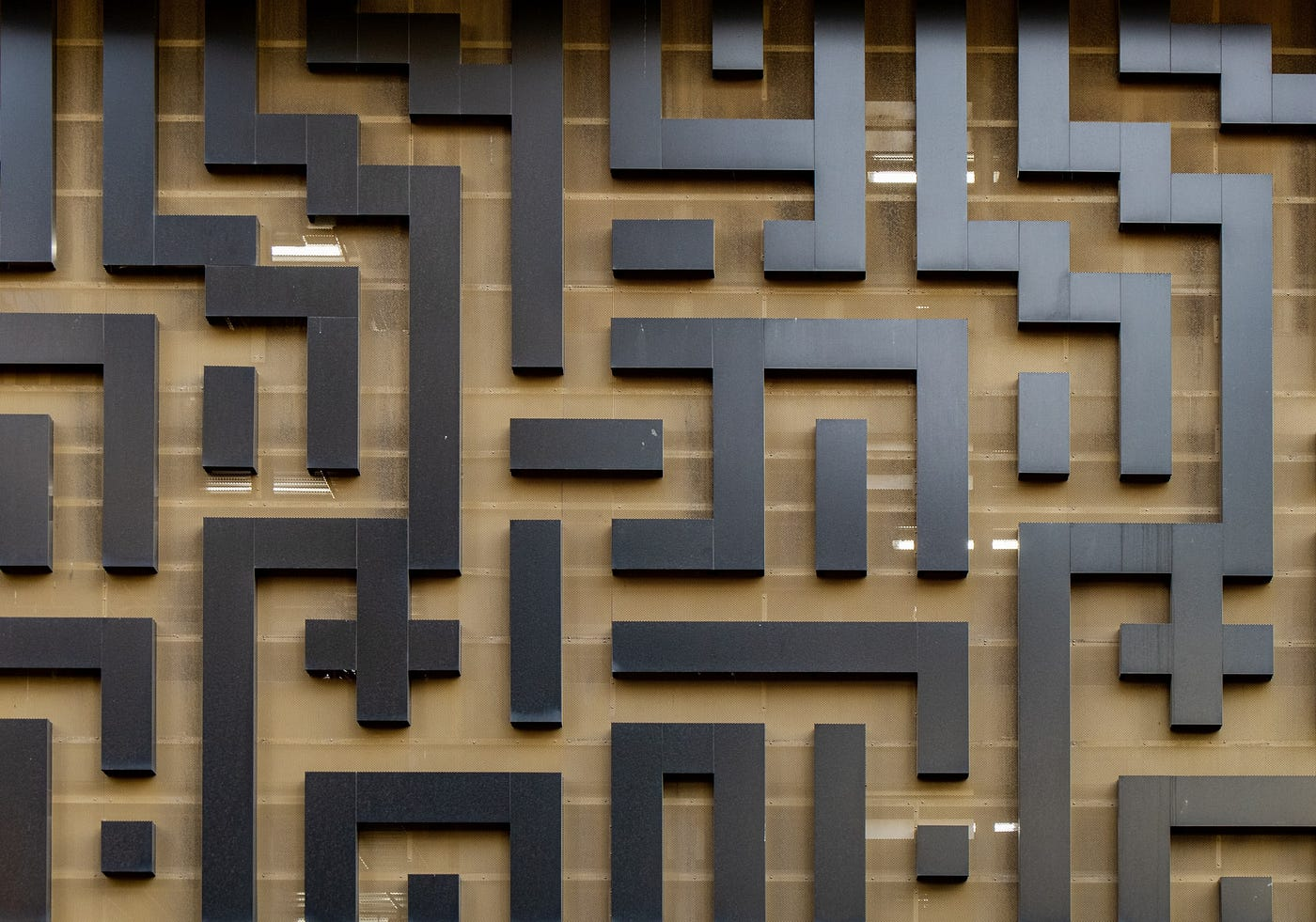Photo by Mitchell Luo on Unsplash

“It’s only forever, not long at all” (David Bowie)

(Curious to see the code? Check it out here! 🧩)

As done in the previous posts, we want to address the problem of generating a maze by starting from an (apparently) unrelated problem: percolation.

Percolation is the movement and filtering of a fluid in a porous material. In particular, if a material is very porous, then the liquid can filter from the surface to the bottom of it; if the material is very dense instead, the liquid spilled on the surface can’t reach the bottom.

Within this context, we can treat the maze as the porous material and a walker as the liquid trying to get to the bottom.

We will write a class “MazeSitePercolationModel” to produce a maze “by (site) percolation” with Python, so first, in the _init__ method, we define a probability p to plug into np.random.binomial to toss a coin over an entire matrix of a chosen size:

`def __init__(self, p, size):  n = 1  self.p = p  self.size = size  self.grid = np.random.binomial(n, self.p, size=(self.size, self.size))`

We will treat ones as blank carved spaces and zeros as walls in the maze.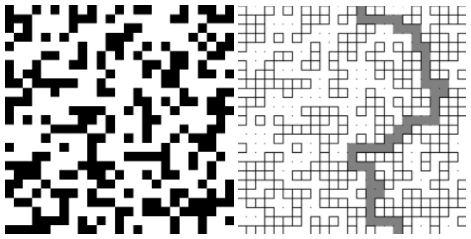Grid generated tossing a coin over a matrix (left) and resulting maze (right)

We also a map of neighbour and a dictionary, containing all the relationships between the roots in the maze and the paths:

`self.neighbours_map = self.build_adjacency_map(self.grid)self.percolation_paths = self.try_to_percolate()`

build_adjancency_map is a method which basically construct an adjacency list for each and every hollow cell in the maze:

In this method, assess_neighbours just tests all the neighbours of a cell i,j in the matrix to satisfy the following assertions:

• being hollow, so that the walker can go there
• being beneath the current cell, because liquid can’t just defy gravity and go up (cells to be evaluated are W,S,E)

try_to_percolate returns a dictionary where all the roots in the maze are keys and a path starting from that root to the bottom of the maze is value.

We define “roots” all the empty cells in the first row and we populate this dictionary as soon as possible, so that it is easy to retrieve a path related to a certain root.

find_percolation_path is just a BFS, returning an eventual path to the end of the maze for the current starting cell:

Those structures and methods are by the way required to understand if the walker “percolates” along the maze.

does_percolate is a function returning a boolean: it checks if a path which leads the walker to an exit exists.

Getting a percolation path from a root is easy as retrieving a value from a dict, given the key:

`def get_percolation_path(self, root) -> list:  return self.percolation_paths[root]`

We can also ask for a random percolation path: we just take one random percolable path from a percolable root; if no percolable root is present, then we return an empty path.

Getting the maze and the path

In a test file we can the following to generate a maze as a more/less porous material:

`pm = maze_percolation.MazeSitePercolationModel(p=0.6, size=25)`

Then we ask ourselves if the maze has a solution (does percolate) and print the maze image as a matrix and as a pretty printed maze.

`does_maze_percolate = pm.does_percolate()pm.output_grid_image('maze_grid.gif')pm.pretty_output_grid_image('maze_percolation.gif',add_walls=False)pm.pretty_output_grid_image('maze_percolation_walls.gif',add_walls=True)`

pretty_output_grid_image is a function printing the maze on a gif image: it accepts a boolean parameter “add_walls”, which outputs the image adding walls to fill the gaps in the maze without altering the does_percolate property.

Adding walls without altering the percolation property

For example: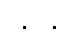Two horizontal dots can, under some circustances, be joined to form a wall

can be easily filled to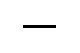A wall from horizontal points joining

and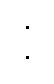Two vertical dots can, under some circustances, be joined to form a wall

can be filled as: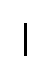A wall from vertical points joining

without actually blocking any of the existing paths.

Adding walls to the currently generated maze leads to the following results: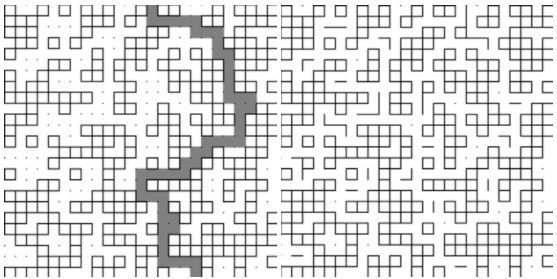A maze with no joined walls (left) and one with walls added (right)

we can notice that entire blocks get added to the maze without changing the percolability results.

If the maze percolates then we randomly get one percolating path and print it to a gif image.

`print('does_maze_percolate: ',does_maze_percolate)rand_perc_path = pm.get_random_percolation_path()pm.output_pretty_percolation_path_image(rand_perc_path,'maze_percolation_path.gif')`

Notable results

As a consequence of the fact that the maze has been generated as a porous material in site percolation (even if with inverted probabilities, being p the probability of an empty space in this case), it shows the same peculiarities.

In particular, getting a maze which can be traversed is extremely difficult for lower probability values and it suddenly gets much more likely to happen around a probability of 0,6 (tipping point).

With a probability of 0.7 it’s almost certain that we are going to get a traversable maze.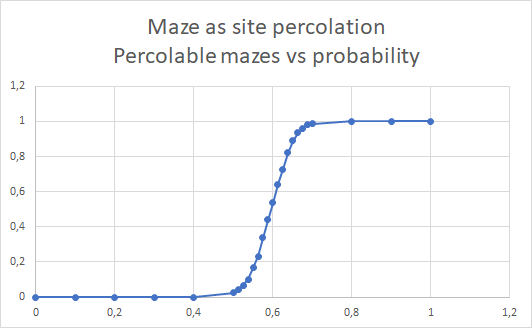Maze by site percolation — number of percolable mazes generated vs generation probability

--

--